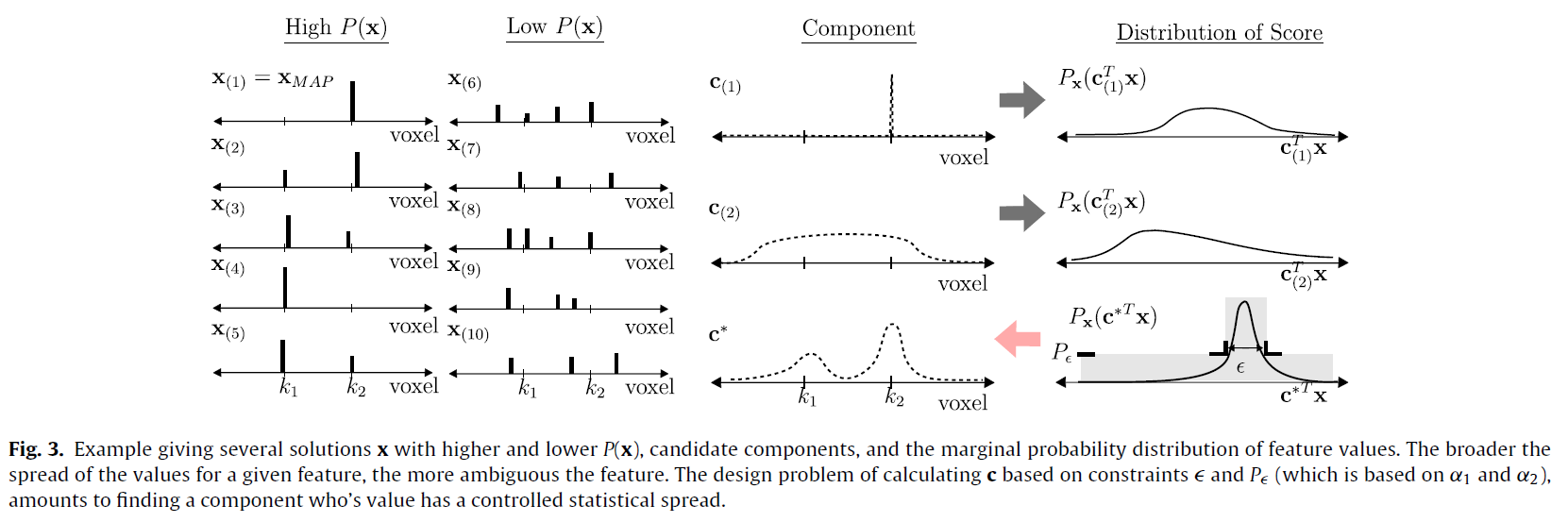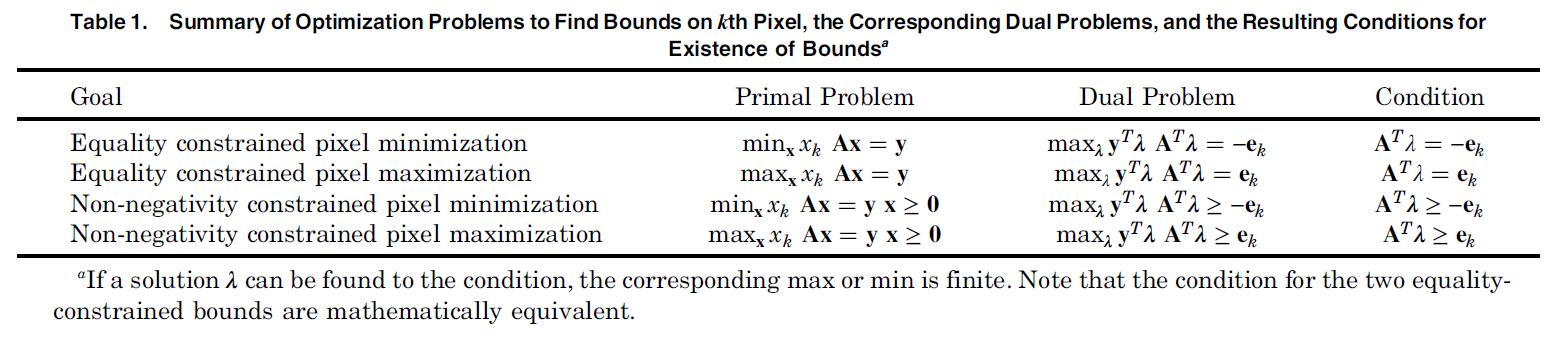## Clustering Gaussian Graphical Models

We derive an efficient method to perform clustering of nodes in Gaussian graphical models directly from sample data. Nodes are clustered based on the similarity of their network neighborhoods, with edge weights defined by partial correlations. In the limited-data scenario, where the covariance matrix would be rank-deficient, we are able to make use of matrix factors, and never need to estimate the actual covariance or precision matrix. We demonstrate the method on functional MRI data from the Human Connectome Project. A matlab implementation of the algorithm is provided.

https://arxiv.org/pdf/1910.02342

## On the Computation and Applications of Large Dense Partial Correlation Networks

While sparse inverse covariance matrices are very popular for modeling network connectivity, the value of the dense solution is often overlooked. In fact the L2-regularized solution has deep connections to a number of important applications to spectral graph theory, dimensionality reduction, and uncertainty quantification. We derive an approach to directly compute the partial correlations based on concepts from inverse problem theory. This approach also leads to new insights on open problems such as model selection and data preprocessing, as well as new approaches which relate the above application areas.

https://arxiv.org/pdf/1903.07181

## Spectral Resolution Clustering for Brain Parcellation

We take an image science perspective on the problem of determining brain network connectivity given functional activity. But adapting the concept of image resolution to this problem, we provide a new perspective on network partitioning for individual brain parcellation. The typical goal here is to determine densely-interconnected subnetworks within a larger network by choosing the best edges to cut. We instead define these subnetworks as resolution cells, where highly-correlated activity within the cells makes edge weights difficult to determine from the data. Subdividing the resolution estimates into disjoint resolution cells via clustering yields a new variation, and new perspective, on spectral clustering. This provides insight and strategies for open questions such as the selection of model order and the optimal choice of preprocessing steps for functional imaging data. The approach is demonstrated using functional imaging data, where we find the proposed approach produces parcellations which are more predictive across multiple scans versus conventional methods, as well as versus alternative forms of spectral clustering.

https://arxiv.org/pdf/1810.04026

## A regularized clustering approach to brain parcellation from functional MRI data

We consider a data-driven approach for the subdivision of an individual subject’s functional Magnetic Resonance Imaging (fMRI) scan into regions of interest, i.e., brain parcellation. The approach is based on a computational technique for calculating resolution from inverse problem theory, which we apply to neighborhood selection for brain connectivity networks. This can be efficiently calculated even for very large images, and explicitly incorporates regularization in the form of spatial smoothing and a noise cutoff. We demonstrate the reproducibility of the method on multiple scans of the same subjects, as well as the variations between subjects.

Keith Dillon, Yu-Ping Wang, ” A regularized clustering approach to brain parcellation from functional MRI data“, Proc. SPIE 10394, Wavelets and Sparsity XVII, 103940E (2017/08/24); doi: 10.1117/12.2274846; http://dx.doi.org/10.1117/12.2274846

## A robust sparse-modeling framework for estimating schizophrenia biomarkers from fMRI

Our goal is to identify the brain regions most relevant to mental illness using neuroimaging. State of the art machine learning methods commonly suffer from repeatability difficulties in this application, particularly when using large and heterogeneous populations for samples. We revisit both dimensionality reduction and sparse modeling, and recast them in a common optimization-based framework. This allows us to combine the benefits of both types of methods in an approach which we call unambiguous components. We use this to estimate the image component with a constrained variability, which is best correlated with the unknown disease mechanism.## Fast and robust estimation of ophthalmic wavefront aberrations

Rapidly rising levels of myopia, particularly in the developing world, have led to an increased need for inexpensive and automated approaches to optometry. A simple and robust technique is provided for estimating major ophthalmic aberrations using a gradient-based wavefront sensor.

## Computational estimation of resolution in reconstruction techniques utilizing sparsity, total variation, and nonnegativity

Techniques which exploit properties such as sparsity and total variation have provided the ability to reconstruct images that surpass the conventional limits of imaging. This leads to difficulties in assessing the result, as conventional metrics for resolution are no longer valid. We develop a numerical approach to evaluating the second-order statistics of the estimate by relating a confidence interval on the solution to a confidence interval on a pixel value, and from this we formulate an approach to estimating the spatial resolution. With this estimate, we can calculate the resolution at each point subject to chosen bounds on the desired precision and confidence.

## Imposing uniqueness to achieve sparsity

In this paper we take a novel approach to the regularization of underdetermined linear systems. Typically, a prior distribution is imposed on the unknown to hopefully force a sparse solution, which often relies on uniqueness of the regularized solution (something which is typically beyond our control) to work as desired. But here we take a direct approach, by imposing the requirement that the system takes on a unique solution. Then we seek a minimal residual for which this uniqueness requirement holds.

## Element-wise uniqueness, prior knowledge, and data-dependent resolution

Techniques for finding regularized solutions to underdetermined linear systems can be viewed as imposing prior knowledge on the unknown vector. The success of modern techniques, which can impose priors such as sparsity and non-negativity, is the result of advances in optimization algorithms to solve problems which lack closed-form solutions. Techniques for characterization and analysis of the system to determine when information is recoverable, however, still typically rely on closed-form solution techniques such as singular value decomposition or a filter cutoff estimate. In this letter we propose optimization approaches to broaden the approach to system characterization.

We start by deriving conditions for when each unknown element of a system admits a unique solution, subject to a broad class of types of prior knowledge. With this approach we can pose a convex optimization problem to find “how unique” each element of the solution is, which may be viewed as a generalization of resolution to incorporate prior knowledge. We find that the result varies with the unknown vector itself, i.e., it is data-dependent, such as when the sparsity of the solution improves the chance it can be uniquely reconstructed. The approach can be used to analyze systems on a case-by-case basis, estimate the amount of important information present in the data, and quantitatively understand the degree to which the regularized solution may be trusted.

K. Dillon and Y. Fainman, “Element-wise uniqueness, prior knowledge, and data-dependent resolution,” SIViP, pp. 1–8, Apr. 2016. (pdf)

## Bounding pixels in computational imaging

We consider computational imaging problems where we have an insufficient number of measurements to uniquely reconstruct the object, resulting in an ill-posed inverse problem. Rather than deal with this via the usual regularization approach, which presumes additional information which may be incorrect, we seek bounds on the pixel values of the reconstructed image.

Formulating the inverse problem as an optimization problem, we find conditions for which a system’s measurements can produce a bounded result for both the linear case and the non-negative case (e.g., intensity imaging). We also consider the problem of selecting measurements to yield the most bounded results. Finally we simulate examples of the application of bounded estimation to different two-dimensional multiview systems.

K. Dillon and Y. Fainman, “Bounding pixels in computational imaging,” Appl. Opt., vol. 52, no. 10, pp. D55–D63, Apr. 2013. (pdf)